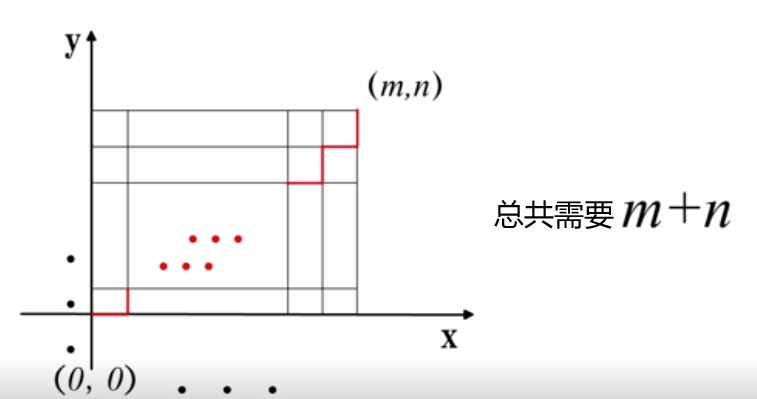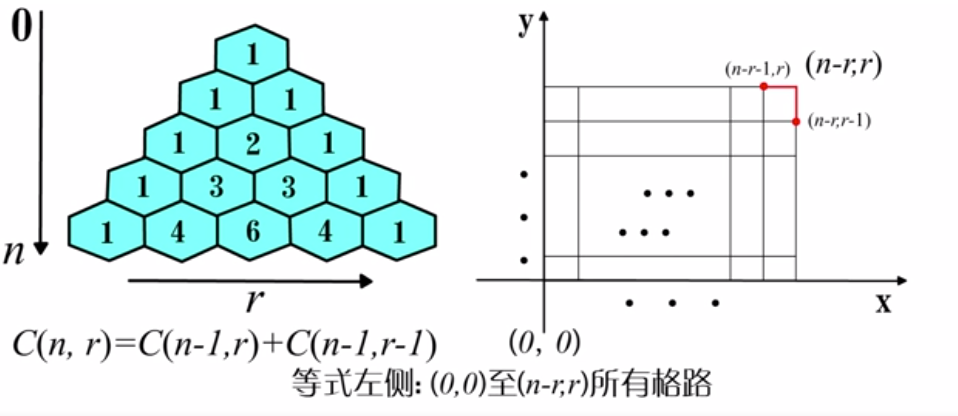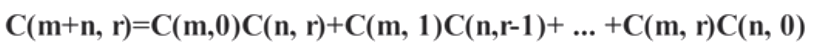# <组合数学>排列组合(1)/格路模型，范德蒙德恒等式

1.排列(permutation)：

从n个不同的元素中，取出r个不重复的元素，按次序排列，称为从n个中取r个的无重排列。

排列的个数用P(n,r)表示或Pr    n>=r       //高中的时候教材教我们Ar，跟这里的一样。

P(n,r) = n!/r!

排列的基本问题是“n个不同球放r个不同盒”问题。

2.组合(conmutation)：

从n个不同的元素中，取出r个不重复的元素组成一个子集而不考虑其元素的顺序，称为从n个中取r个的无重组合。

组合的个数用C(n,r)表示或Crn     n>=r

C(n,r)=n! / [r!*(n-r)!]

组合的基本问题是“n个不同球放r个相同盒”问题。

两个性质：

|—— C(n,r) = C(n,n-r)           //C(8,3)=C(8,5)

|—— C(n,l)*C(l,r) = C(n,r)*C(n-r,l-r) //C(9,5)*C(5,2)=C(9,2)*C(7,3)

3.格路模型与组合恒等式：posted @ 2020-02-10 13:24  dynmi  阅读(766)  评论(0编辑  收藏  举报